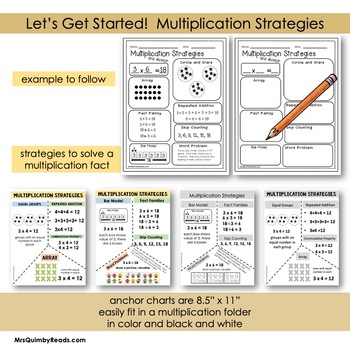# Multiplication | Learn Your Facts | Practice Pages;
3rd - 4th, Homeschool
Subjects
Resource Type
Standards
Formats Included
• PDF
Pages
37 pages
Report this resource to TPT
##### Also included in
1. Learn multiplication facts with activity and practice. It's educational and fun! Strategies, tips and tricks are outlined when learning multiplication. Properties and number sense in multiplication are reviewed. Practice pages with arrays, number bonds, spin and multiply, roll, multiply and color
Price \$58.75Original Price \$80.90Save \$22.15

### Description

Learning Multiplication Facts! Practice pages, strategies, tips and tricks to learn multiplication facts. Properties and number sense in multiplication review. 37 pages for learning, practice, and review. Learning multiplication facts can be fun and easy!

Included in this teaching resource for multiplication:

• Small anchor charts/ explanation pages on strategies to learn for multiplication

• Multiplication Wheels for practice

• Circles and Stars strategy explained and practice pages and directions

• Did you notice? Pages about the times tables that students find very interesting

• Covers for multiplication folders

This math resource is easy to download and print and use for math class and math stations. You can easily place these pages in a math station, make a multiplication folder or notebook.

These worksheets are ideal for whole class practice, small group instruction, independent seat work, use with manipulatives, morning seat work, homework, fast finishers and extra practice. After completing these pages, your students will have a better understanding of the multiplication process. You might also use these pages for independent work practice for multiplication.

There are 37 pages included in this math resource on multiplication practice and review. Thank you for previewing this teaching resource.

Would you like to know when I post new teaching resources? Follow ME on TPT.

Teaching the Language of All Around Numbers & Facts

that Engage Student Thinking:

Roll, Multiply, Write and Color

Spin-Multiply-Color Multiplication

Number Bonds and Arrays- Learn Math Strategies for Multiplication

Multiplication Bar Diagrams

Multiplication Word Problems

Multiplication Facts Assessments and Tracking

Or Save \$ and purchase the bundle: Yes, I want all of them!

Multiplication Bundle

******************************************************************************************

50% off all new teaching resource listings for the first 24 hours. Follow to

******************************************************************************************

Total Pages
37 pages
N/A
Teaching Duration
N/A
Report this resource to TPT
Reported resources will be reviewed by our team. Report this resource to let us know if this resource violates TPT’s content guidelines.

### Standards

to see state-specific standards (only available in the US).
Multiply one-digit whole numbers by multiples of 10 in the range 10–90 (e.g., 9 × 80, 5 × 60) using strategies based on place value and properties of operations.
Interpret products of whole numbers, e.g., interpret 5 × 7 as the total number of objects in 5 groups of 7 objects each. For example, describe a context in which a total number of objects can be expressed as 5 × 7.
Interpret whole-number quotients of whole numbers, e.g., interpret 56 ÷ 8 as the number of objects in each share when 56 objects are partitioned equally into 8 shares, or as a number of shares when 56 objects are partitioned into equal shares of 8 objects each. For example, describe a context in which a number of shares or a number of groups can be expressed as 56 ÷ 8.
Use multiplication and division within 100 to solve word problems in situations involving equal groups, arrays, and measurement quantities, e.g., by using drawings and equations with a symbol for the unknown number to represent the problem.
Determine the unknown whole number in a multiplication or division equation relating three whole numbers. For example, determine the unknown number that makes the equation true in each of the equations 8 × ? = 48, 5 = __ ÷ 3, 6 × 6 = ?.